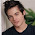## 24 June 2012

### Verilog HDL: Gate Level Modeling

Gate Level Modeling:
à For small number of gate designs;
à Feedbackless structure;
à Used in top level to integrate the design.
Gate –Level Modeling (Structural Modeling):
à mainly includes instantiation of built-in primitives.
à and,nand,or,nor etc

à Design with top-down methodology.
à Implementation with bottom-up methodology.
module half_adder(s,c,a,b)
input a,b;
output s,c;
assign s=a^b;
assign c=a&b;
endmodule

module full_adder(sum,carry,x,y,z)
input x,y,z;
output sum,carry;
half_adder ha1(.s(s1), .c(c1), .a(x), .b(y));
//half_adder ha1(s1,c1,a,b);//above method is good
half_adder ha2(.s(sum), .c(c2), .a(s1), .b(z));
or o1(.c(carry), .a(c1), .b(c2));
endmodule
﻿
module adder4bit(sout,cout,ain,bin,cin)
input [3:0] ain,bin;
input cin;
output [3:0] sout,cout;
full_adder FA1 (.sum(s),.carry(c),.x(a),.y(b),.z(cin));
full_adder FA2 (.sum(s),.carry(c),.x(a),.y(b),.z(c));
full_adder FA3 (.sum(s),.carry(c),.x(a),.y(b),.z(c));
full_adder FA4 (.sum(s),.carry(c),.x(a),.y(b),.z(c));
endmodule

gate level modeling of half Adder:
module halfadder (s,c,a,b)
input a,b;
output s,c;
and a1(c,a,b);     //List of ports
xor x1(s,a,b);      //primitive instatiation; x1 being instatiation label
endmodule;

Gate Level Modelling of Full Adder:
module full_adder(output s, cout, input a,b,cin)
wire s1,cout1,cout2,cout3;
xor x1(s1,a,b);
xor x2(s1,cin);
and a1(cout1,a,b);
and a2(cout2,b,c);
and a3(cout3,c,a);
or o1(cout,cout1,cout2,cout3);
endmodule

Data Flow Modeling:
For small boolean equations; combinational circuits.
module multiplier(port_list)
input IN1, IN2;
output output1;
assign output1=IN1*IN2
endmodule
Half Adder:

module half_adder(s,c,a,b)
input a,b;
output s,c;
assign s=a^b;                     //^ àxor
assign c = a&b;                  //& à and
endmodule
Truth Table For Half Adder:

a              b             s              c
------------------------------------
0              0              0              0
0              1              1              0
1              0              1              0
1              1              0              1
assignà called as continous assign statement; assignment can only to ‘wire’ not for ‘reg’ data type.
The above type of modeling is called “data flow” modelling.

Full –Adder –Data Flow Modelling:
assignà called as continous assign statement; assignment can only to ‘wire’ not for ‘reg’ data type.
The above type of modeling is called “data flow” modelling.

Full –Adder –Data Flow Modelling:
module full_adder(output s, cout, input a, b, cin);
wire s1;
assign s1=a^b;
assign s=s1^cin;
// can also be coded as s=((a^b)^c));
assign cout=(a&b) | (b&c) | (c&a) ;
endmodule
Truth Table For Full Adder:

cin          b             a              s              c
------------------------------------------------
0              0              0              0              0
0              0              1              1              0
0              1              0              1              0
0              1              1              0              1
1              0              0              1              0
1              0              1              0              1
1              1              0              0              1
1              1              1              0              1
Multiplexer:

module mux(z,sel,a,b)
input a,b,sel;
output z;
wire selbar=~sel;
assign z=(a & selbar) | (b & sel);
endmodule

2-Bit Comparator:
module (output g,l,e, input a1,a0,b1,b0)
assign g=({a1,a0}>{b1,b0});
assign l=({a1,a0}<{b1,b0});
assign e=({a1,a0}=={b1,b0});
endmodule

#### 1 comment:

1.This comment has been removed by a blog administrator.

Your Comments... (comments are moderated)